# 用深度学习做命名实体识别(四)——模型训练

ok,下面开始我们的模型训练。

#### 准备训练样本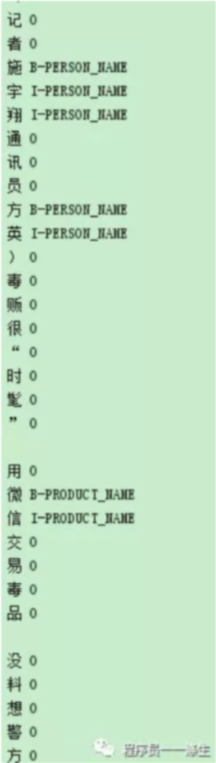• B，即Begin，表示开始
• I，即Intermediate，表示中间
• O，即Other，表示其他，用于标记无关字符

# -*- coding: utf-8 -*-

"""

"""
import codecs
import os

__author__ = '程序员一一涤生'

tag_dic = {"时间": "TIME",
"地点": "LOCATION",
"人名": "PERSON_NAME",
"组织名": "ORG_NAME",
"公司名": "COMPANY_NAME",
"产品名": "PRODUCT_NAME"}

# 转换成可训练的格式，最后以"END O"结尾
def from_ann2dic(r_ann_path, r_txt_path, w_path):
q_dic = {}
print("开始读取文件:%s" % r_ann_path)
with codecs.open(r_ann_path, "r", encoding="utf-8") as f:
line = line.strip("\n\r")
while line != "":
line_arr = line.split()
print(line_arr)
cls = tag_dic[line_arr]
start_index = int(line_arr)
end_index = int(line_arr)
length = end_index - start_index
for r in range(length):
if r == 0:
q_dic[start_index] = ("B-%s" % cls)
else:
q_dic[start_index + r] = ("I-%s" % cls)
line = line.strip("\n\r")

print("开始读取文件:%s" % r_txt_path)
with codecs.open(r_txt_path, "r", encoding="utf-8") as f:
# content_str = content_str.replace("\n", "").replace("\r", "").replace("//////", "\n")
print("开始写入文本%s" % w_path)
with codecs.open(w_path, "w", encoding="utf-8") as w:
for i, str in enumerate(content_str):
if str is " " or str == "" or str == "\n" or str == "\r":
print("===============")
elif str == "/":
if i == len(content_str) - len("//////") + 1:  # 表示到达末尾
# w.write("\n")
break
# 连续六个字符首尾都是/,则表示换一行
elif content_str[i + len("//////") - 1] == "/" and content_str[i + len("//////") - 2] == "/" and \
content_str[i + len("//////") - 3] == "/" and content_str[i + len("//////") - 4] == "/" and \
content_str[i + len("//////") - 5] == "/":
w.write("\n")
i += len("//////")
else:
if i in q_dic:
tag = q_dic[i]
else:
tag = "O"  # 大写字母O
w.write('%s %s\n' % (str, tag))
w.write('%s\n' % "END O")

# 去除空行
def drop_null_row(r_path, w_path):
q_list = []
with codecs.open(r_path, "r", encoding="utf-8") as f:
line = line.strip("\n\r")
while line != "END O":
if line != "":
q_list.append(line)
line = line.strip("\n\r")
with codecs.open(w_path, "w", encoding="utf-8") as w:
for i, line in enumerate(q_list):
w.write('%s\n' % line)

# 生成train.txt、dev.txt、test.txt
# 除8，9-new.txt分别用于dev和test外,剩下的合并成train.txt
def rw0(data_root_dir, w_path):
if os.path.exists(w_path):
os.remove(w_path)
for file in os.listdir(data_root_dir):
path = os.path.join(data_root_dir, file)
if file.endswith("8-new.txt"):
# 重命名为dev.txt
os.rename(path, os.path.join(data_root_dir, "dev.txt"))
continue
if file.endswith("9-new.txt"):
# 重命名为test.txt
os.rename(path, os.path.join(data_root_dir, "test.txt"))
continue
q_list = []
print("开始读取文件:%s" % file)
with codecs.open(path, "r", encoding="utf-8") as f:
line = line.strip("\n\r")
while line != "END O":
q_list.append(line)
line = line.strip("\n\r")
print("开始写入文本%s" % w_path)
with codecs.open(w_path, "a", encoding="utf-8") as f:
for item in q_list:
if item.__contains__('\ufeff1'):
print("===============")
f.write('%s\n' % item)

if __name__ == '__main__':
data_dir = "datas"
for file in os.listdir(data_dir):
if file.find(".") == -1:
continue
file_name = file[0:file.find(".")]
r_ann_path = os.path.join(data_dir, "%s.ann" % file_name)
r_txt_path = os.path.join(data_dir, "%s.txt" % file_name)
w_path = "%s/new/%s-new.txt" % (data_dir, file_name)
from_ann2dic(r_ann_path, r_txt_path, w_path)
# 生成train.txt、dev.txt、test.txt
rw0("%s/new" % data_dir, "%s/new/train.txt" % data_dir)

ok，到此我们的训练数据就准备好了，接下来我们需要准备预训练模型。

#### 准备预训练模型

bert在中文维基百科上预训练的模型下载地址：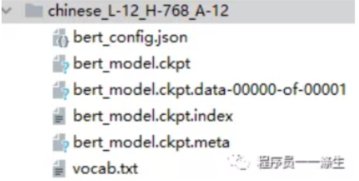#### 训练

https://github.com/macanv/BERT-BiLSTM-CRF-NER

• 原来的项目是采用install的方式直接将项目安装到你的python虚拟环境下，然后通过命令行执行训练，笔者直接调整了源代码，为了可以基于源代码执行一些调试；
• 原来的项目训练的时候几乎没有日志信息，修改后的项目可以看到训练日志；
• 原来的项目只能在训练结束后输出评估结果，修改后的项目可以让评估脱离训练过程独立进行。

1. 将之前下载的bert预训练模型chinese_L-12_H-768_A-12目录以及目录中的文件放到项目的models目录下。
2. 将之前准备的train、dev、test三个文件放到person_data目录下。
3. 为该项目新建一个python的虚拟环境，然后安装所需要的依赖包，关于需要哪些依赖包，项目中的requirement.txt是这么描述的：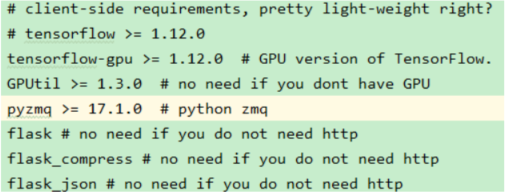tensorflow的安装，因为我们是在GPU上训练，所以只需要安装tensorflow-gpu，笔者安装的是tensorflow1.13.1版本，因为笔者的CUDA版本是10.0。

nohup python bert_lstm_ner.py -max_seq_length 500 -batch_size 2 -learning_rate 2e-5 -num_train_epochs 3.0 -filter_adam_var True -verbose -data_dir person_data -output_dir output -init_checkpoint models/chinese_L-12_H-768_A-12/bert_model.ckpt -bert_config_file models/chinese_L-12_H-768_A-12/bert_config.json -vocab_file models/chinese_L-12_H-768_A-12/vocab.txt >log.out 2>&1 &

• nohup
使用nohup命令，可以保证在命令窗口被关闭，或远程链接中断的情况下，不影响远端python程序的执行。python程序执行过程中的日志信息会保存在当前文件夹下的log.out文件中。
• max_seq_length
每个样本的最大长度，不能超过512。如果你的某些样本超过了这个长度，需要截断。截断代码可以使用项目根路径下的data_process.py文件。
• batch_size
每次送到模型进行训练的样本数量。一般是2幂次方。如果你的GPU显存够大，可以尝试增大batch_size。
• learning_rate
初始学习率，用于调整模型的学习速度，过大过小都不好。刚开始训练时：学习率以 0.01 ~ 0.001 为宜。接近训练结束：学习速率的衰减应该在100倍以上。这里因为我们采用的是迁移学习，由于预模型本身已经在原始数据集上收敛，此时学习率应该设置的较小，所以这里设置成0.00002。
• num_train_epochs
每次用完所有样本后，记为一个epoch。这里是指设置多少个epoch后训练结束。
• verbose
加上该参数就会打开tensorflow的日志。
• data_dir
train、dev、test数据所在的目录。
• output_dir
模型输出目录。
• init_checkpoint
预训练模型的路径，这里我们使用了bert的中文预训练模型。
• bert_config_file
bert模型的配置文件所在路径。
• vocab_file
bert的词汇表文件路径。

tail -f log.out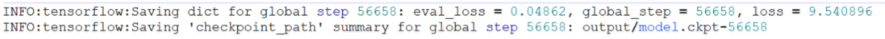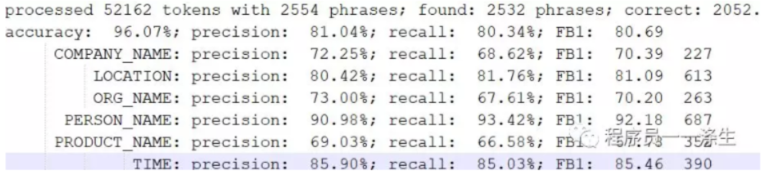#### 测试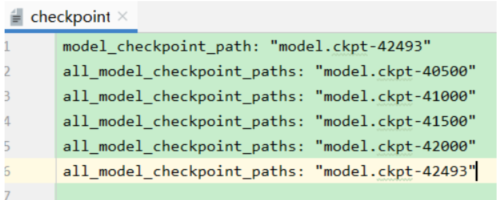python bert_lstm_ner-test.py

ok，本篇就这么多内容啦~，感谢阅读O(∩_∩)O，88~posted @ 2019-09-09 18:01  程序员一一涤生  阅读(...)  评论(...编辑  收藏